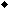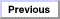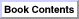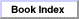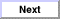Inside Macintosh: AppleScript Language Guide / Part 2 - AppleScript Language Reference
Chapter 6 - Expressions / Operations
Operators That Handle Operands of Various Classes

### Equal, Is Not Equal To

The Equal and Is Not Equal To operators can handle operands of any class.

##### OPERANDS OF DIFFERENT CLASSES
Two expressions of different classes are not equal.

##### BOOLEAN EXPRESSION
Two Boolean expressions are equal if both of them evaluate to `true` or if
both evaluate to `false`. They are not equal if one evaluates to `true` and the other to `false`.

##### CLASS IDENTIFIER
Two class identifiers are equal if they are the same identifier. They are not equal if they are different identifiers.

##### CONSTANT
Two constants are equal if they are the same. They are not equal if they
are different.

##### DATA
Two data values are equal if they are the same length in bytes and their bytes are the same (AppleScript does a byte-wise comparison).

##### DATE
Two dates are equal if they both represent the same date, even if they are expressed in different formats. For example, the following expression is `true`, because `date "12/5/92"` and `date "December 5th, 1992"` represent the same date.

```date "12/5/92" = date "December 5th, 1992"
```
##### INTEGER
Two integers are equal if they are the same. They are not equal if they
are different.

##### LIST
Two lists are equal if each item in the list to the left of the operator is equal to the item in the same position in the list to the right of the operator. They are not equal if items in the same positions in the lists are not equal or if the lists have different numbers of items. For example,

```{ (1 + 1), (4 > 3) } = {2, true}
```
is `true`, because `(1 + 1)` evaluates to `2`, and `(4 > 3)` evaluates to `true`.

##### REAL
Two real numbers are equal if they both represent the same real number, even if the formats in which they are expressed are different. For example, the following expression is `true`.

```0.01 is equal to 1e10-2
```
Two real numbers are not equal if they represent different real numbers.

##### RECORDS
Two records are equal if they both contain the same collection of properties and if the values of properties with the same labels are equal. They are not equal if the records contain different collections of properties, or if the values of properties with the same labels are not equal. The order in which properties are listed does not affect equality. For example, the following expression is `true`.

```{ name:"Eric", mileage:"8000" } = { mileage:"8000",ÿ
name:"Eric"}
```
##### REFERENCE
Two references are equal if their classes, reference forms, and containers are identical. They are not equal if their classes, reference forms, and containers are not identical, even if they refer to the same object.

For example, the expression `x = y` in the following Tell statement is `true`, because the classes (`word`), reference forms (Index), and containers (`paragraph 1 of document "Intro" of application "Scriptable Text Editor"`) of the two references are identical.

```tell document "Intro" of application  ÿ
"Scriptable Text Editor"
set x to a reference to word 1 of paragraph 1
set y to a reference to word 1 of paragraph 1
x = y
end tell
--result:true
```
The expression `x = y` in the following statement is `false`, because the containers are different.

```tell document "Intro" of application ÿ
"Scriptable Text Editor"
set x to a reference to word 1 of paragraph 1
set y to a reference to word 1
x = y
end tell
--result:false
```
When you use references in expressions without the A Reference To operator, the values of the objects specified in the references are used to evaluate the expressions. For example, the result of the following expression is `true` if both documents begin with the same word.

```word 1 of document "Report" = word 1 document "Intro"
```
##### STRING
Two strings are equal if they are both the same series of characters. They are not equal if they are different series of characters. AppleScript compares strings character by character. It does not distinguish uppercase from lowercase letters unless you use a Considering statement to consider the `case` attribute. For example, the following expression is `true`.

```"DUMPtruck" is equal to "dumptruck"
```
AppleScript considers all characters and punctuation, including spaces, tabs, return characters, diacritical marks, hyphens, periods, commas, question marks, semicolons, colons, exclamation points, backslash characters, and single and double quotation marks in string comparisons. AppleScript ignores style in string comparisons.

Note
All string comparisons can be affected by Considering and Ignoring statements, which allow you to selectively consider or ignore the case of characters, as well as specific types of characters. For more information, see "Considering and Ignoring Statements" on page 213.